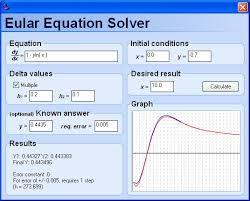# Expression 3350

Calculate the value of the expression x / y, if you know that
(-4x + 3y) / y = 5

k =  -0.5

### Step-by-step explanation:

-4k + 3 = 5

-4·k + 3 = 5

4k = -2

k = -2/4 = -0.5

k = -1/2 = -0.5

Our simple equation calculator calculates it.Did you find an error or inaccuracy? Feel free to write us. Thank you!

Tips for related online calculators
Do you have a linear equation or system of equations and looking for its solution? Or do you have a quadratic equation?